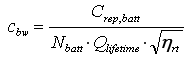# HOMER Pro 3.9Type: Intermediate Variable Units: \$/kWh Symbol: cbw

The storage wear cost is the cost of cycling energy through the storage bank. If the storage properties indicate that the storage life is limited by throughput, then HOMER assumes the storage bank will require replacement once its total throughput equals its lifetime throughput. Each kWh of throughput therefore brings the storage bank that much closer to needing replacement. HOMER calculates the storage wear cost using the following equation:where: Crep,batt = replacement cost of the storage bank [\$] Nbatt = the number of batteries in the storage bank Qlifetime = the lifetime throughput of a single storage [kWh] ηrt = storage roundtrip efficiency [fractional]

The storage bank's marginal cost of generation is equal to the sum of the storage wear cost and the storage energy cost.